Update: 2016-08-17 09:31 PM -0400

# Speaking Mon-Myan Language

spk-all-3.htm

by U Kyaw Tun (UKT) (M.S., I.P.S.T., USA), Daw Khin Wutyi, and staff of Tun Institute of Learning (TIL).
from Learn Mon Yourself : pronouncing all 61 lessons (Spk-all):
Downloaded and set in HTML by UKT and staff of TIL . Not for sale. No copyright. Free for everyone. Prepared for students and staff of TIL  Computing and Language Center, Yangon, MYANMAR :  http://www.tuninst.net , www.romabama.blogspot.com

UKT151018: Original Video & SND for each lesson in Lesson groups are in the TIL SD-Library, and you will not hear them unless you are on a TIL computer.

SpkAll group #03
Speak All Lesson group#03, lessons16-28
Lesson 16 :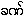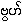Lesson 17 :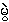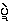Lesson 18 :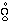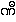Lesson 19
Lesson 20
Lesson 21
Lesson 22
Lesson 23
Lesson 24
Lesson 25
Lesson 26
Lesson 27
Lesson 28

## Lesson 16

SN-SpkAll-les16-61<Ô> / bkp16<Ô> (link chk 160810), & lesson16-61<))
There was no distinct cap. Treat lesson16-61<)) as txt.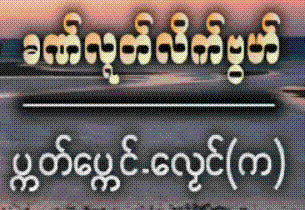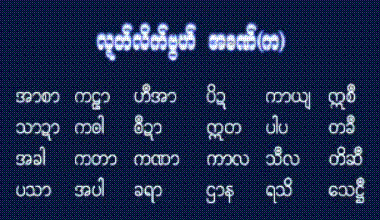Go vertical. Treat lesson16-61<)) as txt.

## Lesson 17

SN-SpkAll-les17-61<Ô> / bkp17<Ô> (link chk 160810), & lesson17-61<))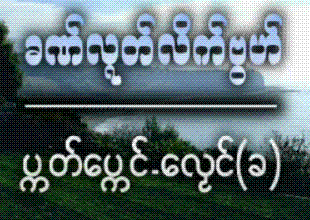lesson17-61cap<))

Pronunciation ofRemember Monipa (is pronounced as)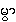{mwa.}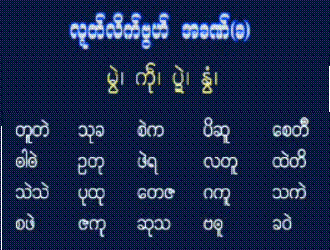Go vertical. - lesson17-61txt<))

## Lesson 18

SN-SpkAll-les18-61<Ô> / bkp18<Ô> (link chk 160810), & lesson18-61<))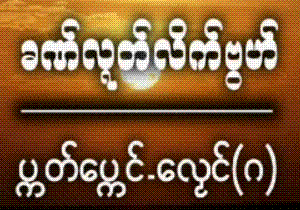lesson18-61cap<))

Pronunciation ofGo vertical. - lesson18-61txt<))

## Lesson 19

SN-SpkAll-les19-61<Ô> / bkp19<Ô> (link chk 160810), & lesson19-61<))
There was no distinct cap. Treat lesson19-61<)) as txt.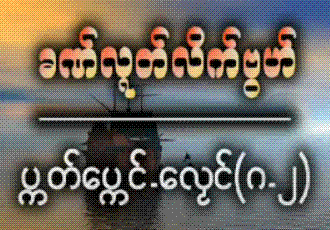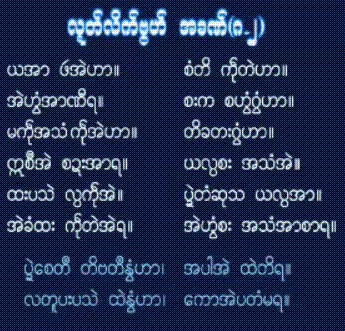Go vertical for white, and horizontal for blue. Treat lesson19-61<)) as txt.

## Lesson 20

SN-SpkAll-les20-61<Ô> / bkp20<Ô> (link chk 160810), & lesson20-61<))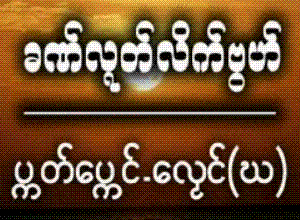- lesson20-61cap<))

Pronunciation of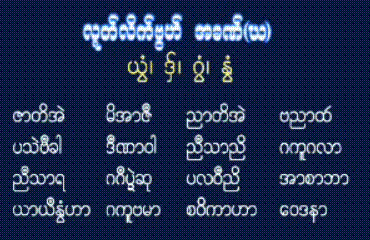Go vertical. lesson20-61txt<))

## Lesson 21

SN-SpkAll-les21-61<Ô> / bkp21<Ô> (link chk 160810), & lesson21-61<))
There was no distinct cap. Treat lesson21-61<)) as txt.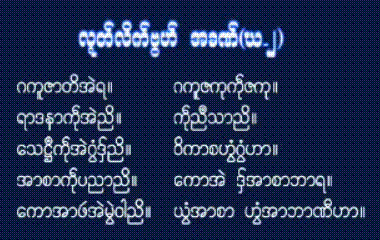Go vertical. Treat lesson21-61<)) as txt.

## Lesson 22

SN-SpkAll-les22-61<Ô> / bkp22<Ô> (link chk 160811), &  lesson22-61<))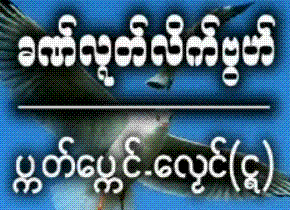lesson22-61cap<))

Pronunciation of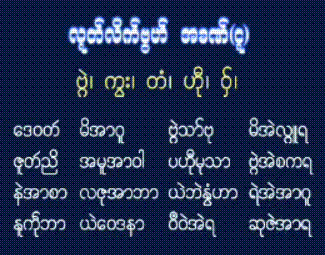Go vertical. - lesson22-61txt<))

## Lesson 23

SN-SpkAll-les23-61<Ô> / bkp23<Ô> (link chk 160811), & lesson23-61<))
There was no distinct cap. Treat lesson23-61<)) as txt.
UKT 130411: The original sound file was not complete.
Only 4 entries could be obtained.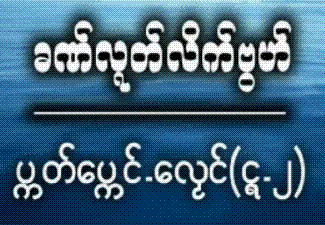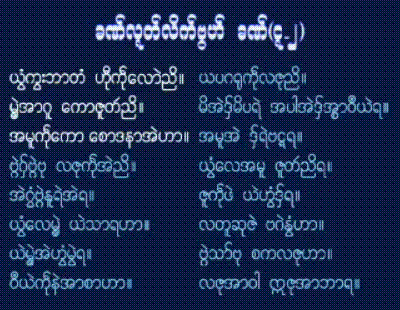Go vertical. Treat lesson23-61<)) as txt.

## Lesson 24

SN-SpkAll-les24-61<Ô> / bkp24<Ô> (link chk 160811), & lesson24-61<))

UKT 130411: This file came with an extension.- lesson24-61cap<))

Listen to Lesson - lesson24-61cap<)) .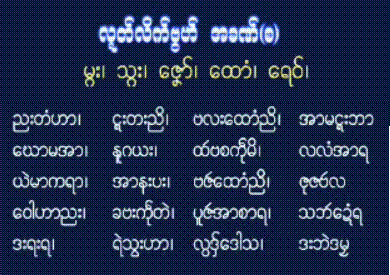Go vertical - lesson24-61txt<))## Lesson 25<))

SN-SpkAll-les25-61<Ô> / bkp25<Ô> (link chk 160811), & lesson25-61<))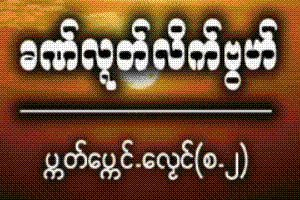Listen to Lesson 25 - lesson25-61<)) .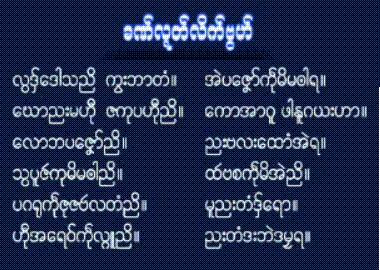Go vertical.

## Lesson 26<))

SN-SpkAll-les26-61<Ô> / bkp26<Ô> (link chk 160811), & lesson26-61<))- lesson26-61cap<))Go vertical - lesson26-61txt<))

## Lesson 27<))

SN-SpkAll-les27-61<Ô> / bkp27<Ô> (link chk 160811), & lesson27-61<))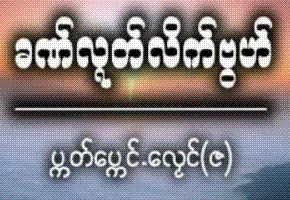- lesson27-61cap<))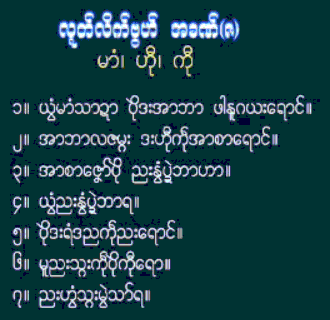- lesson27-61txt<))

## Lesson 28<))

SN-SpkAll-les28-61<Ô> / bkp28<Ô> (link chk 160811), & lesson28-61<))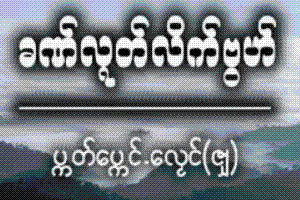lesson28-61cap<))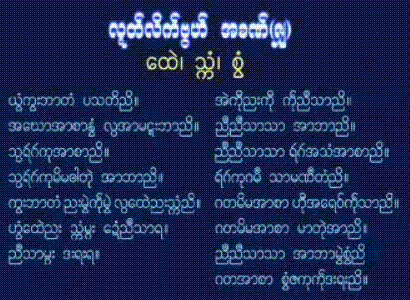lesson28-61txt<))

End TIL file